2016-07-11 15:56:22 chengkangchengkang 阅读数 1046
• ###### 学习OpenCV3.2+QT5+ffmpeg实战开发视频编辑器视频教程

OpenCV3.2+QT5+ffmpeg实战开发视频编辑器视频培训课程概况：教程中会讲解到基于opencv视频和摄像机录制、播放和播放进度控制，多视频图像合并、多视频图像融合、剪切、视频亮度、对比度、尺寸（近邻插值（手动实现），双线性插值，图像金字塔）、颜色格式（灰度图，二值化（阈值）），旋转镜像，视频裁剪（ROI），视频水印（ROI+weight），导出处理后的视频（包含音频，使用ffmpeg工具对音频进行抽取、剪切和终于opencv处理的视频合并）。

19572 人正在学习 去看看 夏曹俊
#include<opencv2/core/core.hpp>
#include<opencv2/highgui/highgui.hpp>
#include<opencv2/opencv.hpp>
#include"iostream"

using namespace std;
using namespace cv;
class Histogram1D
{
private:
int histSize;//the number of
float hranges;//the maximum and min of the pixel
const float*ranges;
int channels;//only use one channel
public:
Histogram1D(){
// the parementer of the 1D histogram
histSize=256;
hranges=0.0;
hranges=255.0;
ranges=hranges;
channels=0;//the normal
}
//calculate the distribution of the 1d
cv::MatND getHistogram(const cv::Mat &image)
{
cv::MatND hist;
//calculate the histogram
cv::calcHist(&image,
1,//calculate the single image's histogram
channels,//the number of channels
cv::Mat(),// not use the image as mask;
hist,//return hist
1,//one-di
histSize,//the number of
ranges//the range of pixel
);
return hist;
}
cv::Mat getHistogramImage(const cv::Mat &image)
{
//first ,calculate the histogram
cv::MatND hist = getHistogram(image);
//receive the max and min number
double maxVal = 0;
double minVal = 0;
cv::minMaxLoc(hist,&minVal,&maxVal,0,0);
//imshow the image of histogram
cv::Mat histImg(histSize,histSize,
CV_8U,cv::Scalar(255));
//set up the highest point is the 90 precent of the nbins
int hpt = static_cast<int>(0.9*histSize);
// every level,draw a vertical line
for (int h =0;h<histSize;h++)
{
float binVal = hist.at<float>(h);
int intensity = static_cast<int>(binVal*hpt/maxVal);
//draw a line with two point
cv::line(histImg,cv::Point(h,histSize),
cv::Point(h,histSize-intensity),
cv::Scalar::all(0));
}
return histImg;
}
};
int main()
{
// the histogram
Histogram1D h;
//calculate hist
cv::MatND histo=h.getHistogram(image);
//scan the every level
for(int i=0;i<256;i++)
cout<<"Value"<<i<<"="<<
histo.at<float>(i)<<endl;
cv::namedWindow("Histogram");
cv::imshow("Histogram",
h.getHistogramImage(image));
cv::Mat thresholded;
cv::threshold(image,thresholded,80,255,cv::THRESH_BINARY);
cv::namedWindow("binary image");
cv::imshow("binary image",thresholded);
cv::waitKey(0);
system("pause");
return 0;
}

2019-04-05 10:01:38 AlonewaitingNew 阅读数 1193
• ###### 学习OpenCV3.2+QT5+ffmpeg实战开发视频编辑器视频教程

OpenCV3.2+QT5+ffmpeg实战开发视频编辑器视频培训课程概况：教程中会讲解到基于opencv视频和摄像机录制、播放和播放进度控制，多视频图像合并、多视频图像融合、剪切、视频亮度、对比度、尺寸（近邻插值（手动实现），双线性插值，图像金字塔）、颜色格式（灰度图，二值化（阈值）），旋转镜像，视频裁剪（ROI），视频水印（ROI+weight），导出处理后的视频（包含音频，使用ffmpeg工具对音频进行抽取、剪切和终于opencv处理的视频合并）。

19572 人正在学习 去看看 夏曹俊

# 灰度直方图原理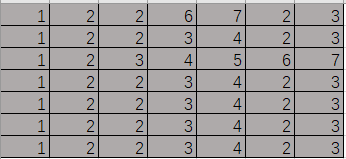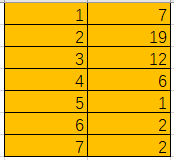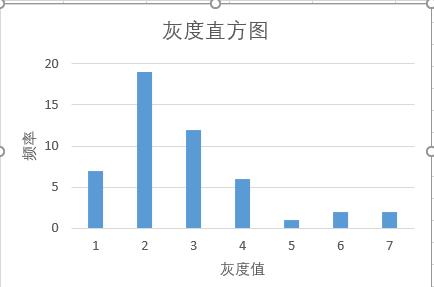1.可以为阈值分割提供一定的依据。
2.灰度直方图均值化提高灰度图像的对比度
3.反向投影直方图检测特定图像的内容
4.均值平移算法可以检测目标的移动
5.灰度直方图的对比，可以寻找相似图片，为图片分类，提供一定的依据

## 灰度直方图实现

``````#pragma once
#include "opencv2/core/core.hpp"
#include "opencv2/imgproc/imgproc.hpp"
#include "opencv2/calib3d/calib3d.hpp"
#include "opencv2/highgui/highgui.hpp"
#include <iostream>
#include <string>
#include <fstream>
using namespace std;
using namespace cv;
class Histogram1D {
private:
int histSize;//定义灰度直方图中类型的数目
float hrange;//定义灰度直方图值的范围
const float * ranges;//定义一个指针数组
int channels;//定义检查的通道数
public:
Histogram1D() {
histSize = 256;
hrange = 0;
hrange = 256;
ranges = hrange;
channels = 0;
}
//获取灰度直方图各个类型的频率
Mat getHistogram(const Mat &image) {
Mat hist;
calcHist(&image,1,channels,Mat(),hist,1,histSize,ranges);
return hist;
}
//画图函数
static Mat getImageOfHistogram(const Mat &hist, int zoom)
{
double maxval = 0.0;
double minval = 0.0;
minMaxLoc(hist, &minval, &maxval, 0, 0);
int histSize = hist.rows;
Mat histImg(histSize*zoom, histSize*zoom, CV_8U, Scalar(255));
int hpt = static_cast<int>(0.9*histSize);
for (int h = 0;h < histSize;h++)
{
float binVal = hist.at<float>(h);
if (binVal > 0)
{
int intensity = (int)(binVal*hpt / maxval);
line(histImg, Point(h*zoom, histSize*zoom), Point(h*zoom, (histSize - intensity)*zoom), Scalar(0), zoom);
}
}
return histImg;
}
Mat getHistogramImage(const Mat &image, int zoom = 1)
{
Mat hist = getHistogram(image);
return getImageOfHistogram(hist, zoom);
}
};
{
imshow("nomal", image1);
imshow("gray", image);
Histogram1D h;
Mat hist = h.getHistogram(image);

//将二值图与图的形式展现
Mat img = h.getHistogramImage(image);

//寻找占比例最大的灰度
int index = 0;
float flag = hist.at<float>(0);
for (int i = 0;i < 256;i++)
{
if (hist.at<float>(i)>flag)
{
flag = hist.at<float>(i);
index = i;
}
//cout << "value" << i << "=" << hist.at<float>(i) << endl;
}
cout << "the max value of the Histgram is:" << index << endl;

namedWindow("Histgram", WINDOW_AUTOSIZE);
imshow("Histgram", img);

Mat thresh;
threshold(image, thresh, index, 255, THRESH_BINARY);
namedWindow("thresh", WINDOW_AUTOSIZE);
imshow("thresh", thresh);

waitKey(0);
//cout << "hello world" << endl;
return 0;
}
int main()
{
system("pause");
return 0;
}
``````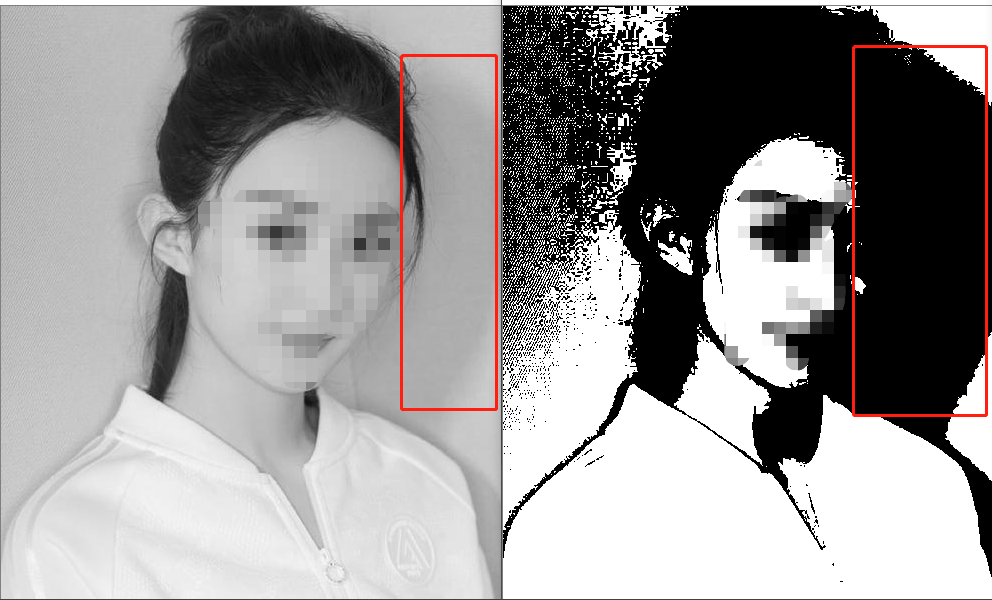这是根据直方图的分布而选的阈值做的阈值分割结果，可以看到该阈值能够较好的分割图像，图像的边缘基本能够找到，这为下一步图像的边缘提取，图像的形态学处理打下了基础。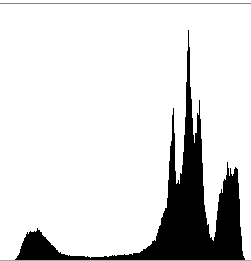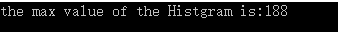## 小结

1.可以为阈值分割提供一定的依据。
2.灰度直方图均值化提高灰度图像的对比度
3.反向投影直方图检测特定图像的内容
4.均值平移算法可以检测目标的移动
5.灰度直方图的对比，可以寻找相似图片，为图片分类，提供一定的依据

2014-07-02 22:58:29 u012771236 阅读数 2719
• ###### 学习OpenCV3.2+QT5+ffmpeg实战开发视频编辑器视频教程

OpenCV3.2+QT5+ffmpeg实战开发视频编辑器视频培训课程概况：教程中会讲解到基于opencv视频和摄像机录制、播放和播放进度控制，多视频图像合并、多视频图像融合、剪切、视频亮度、对比度、尺寸（近邻插值（手动实现），双线性插值，图像金字塔）、颜色格式（灰度图，二值化（阈值）），旋转镜像，视频裁剪（ROI），视频水印（ROI+weight），导出处理后的视频（包含音频，使用ffmpeg工具对音频进行抽取、剪切和终于opencv处理的视频合并）。

19572 人正在学习 去看看 夏曹俊

# 灰度直方图

• 生成并绘制直方图：
```src = imread('Fig0303(a)(breast).tif');
imshow(src);
h1 = imhist(src);                      %生成直方图
figure,imhist(src,255);             %绘制直方图

h2 = imhist(src, 25);                 %生成直方图
horz = linspace(0, 255, 25);
figure,bar(horz, h2);                 %绘制条状直方图
axis([0 255 0 60000]);               %设置坐标轴最大最小值
set(gca, 'xtick', 0:50:250);          %设置坐标轴的标尺
set(gca, 'ytick', 0:20000:60000);
xlabel('灰度级', 'fontsize', 10);  %坐标轴附加图像
ylabel('灰度值', 'fontsize', 10);
text(150, 40000, '条形直方图', 'fontsize',15);

h3 = imhist(src, 25);                 %生成直方图
horz = linspace(0, 255, 25);
figure,stem(horz, h3, 'fill');      %绘制杆状直方图
axis([0 255 0 60000]);               %设置坐标轴最大最小值
set(gca, 'xtick', 0:50:250);         %设置坐标轴的标尺
set(gca, 'ytick', 0:20000:60000);
xlabel('灰度级', 'fontsize', 10); %坐标轴附加图像
ylabel('灰度值', 'fontsize', 10);
text(150, 40000, '杆状直方图', 'fontsize',15);

h4 = imhist(src);                       %生成直方图
figure,plot(h4);                         %绘连续直方图
xlim('auto');                              %自动设置坐标轴范围和标尺
ylim('auto');
text(150, 40000, '连续直方图', 'fontsize',15);```• 直方图均衡化（可使图像的灰度间距拉开或使灰度分布均匀）：
```src = imread('Fig0303(a)(breast).tif');
result = histeq(src);    %均衡化
imshow(result);```• 自适应直方图均衡化：
```src = imread('Fig0303(a)(breast).tif');
figure, imshow(src);
figure, imhist(src);
figure, imshow(result);
figure, imhist(result);```• MATLAB函数小结：
imhist( )：该函数用于获取图像数据直方图。（PS：若没有赋值给左边的变量，该函数将显示一张灰度直方图）
h1 = imhist( src, n )
src--输入图像
n   --灰度级别，默认为256

linspace(a, b, n)：该函数生成一个(1xn)数组，数据的第一个元素值为a，最后一个元素为b，n是总采样点数。（PS：所有元素的值均匀分布）

bar(horz, h2)：绘制条状直方图，通过horz控制直方图显示的密集程度
horz -- 一个(1xn)数组（跟h2同阶）
h2    -- 要绘制的直方图

stem(horz, h3, 'fill')：绘制杆状直方图
horz -- 一个(1xn)数组（跟h2同阶）
h2    -- 要绘制的直方图
'fill'  -- 杆头是否空心

plot(h4)：绘连续直方图

result = histeq(src);    %获取直方图均衡化后的图像

2015-12-01 21:47:40 GarfieldEr007 阅读数 9581
• ###### 学习OpenCV3.2+QT5+ffmpeg实战开发视频编辑器视频教程

OpenCV3.2+QT5+ffmpeg实战开发视频编辑器视频培训课程概况：教程中会讲解到基于opencv视频和摄像机录制、播放和播放进度控制，多视频图像合并、多视频图像融合、剪切、视频亮度、对比度、尺寸（近邻插值（手动实现），双线性插值，图像金字塔）、颜色格式（灰度图，二值化（阈值）），旋转镜像，视频裁剪（ROI），视频水印（ROI+weight），导出处理后的视频（包含音频，使用ffmpeg工具对音频进行抽取、剪切和终于opencv处理的视频合并）。

19572 人正在学习 去看看 夏曹俊

1.    灰度直方图

2.    直方图均衡化

3.    直方图规范化

4.    色彩直方图

1.灰度直方图从概率的观点来理解，灰度出现的频率可看作其出现的概率，这样直方图就对应于概率密度函数pdf(probability density function)，而概率分布函数就是直方图的累积和，即概率密度函数的积分，如下图所示：1.    初始化 hist[k]=0 ; k=0,…,L-1

2.    统计 hist[f(x,y)]++ ; xy =0,…,M-1, 0,…,N-1

3.    标准化 hist[f(x,y)]/=M*N

2.直方图均衡化

直方图均衡化是通过灰度变换将一幅图象转换为另一幅具有均衡直方图，即在每个灰度级上都具有相同的象素点数的过程。3.直方图规范化4.色彩直方图

色彩直方图是高维直方图的特例，它统计色彩的出现频率，即色彩的概率分布信息。通常这需要一定的量化过程，将色彩分成若干互不重叠的种类。一般不直接在RGB色彩空间中统计，而是在将亮度分离出来后，对代表色彩部分的信息进行统计，如在HSI空间的HS子空间、YUV空间的UV子空间，以及其它反映人类视觉特点的彩色空间表示中进行。例如，下图是统计肤色分布情况的例子。（图片来源：Lv Fengjun, Ai Haizhou, et.al., Face Detection Based on Skin Color and Template Matching, ICIG'2000, Aug.16-18, 2000.）

1.编制直方图均衡化及直方图规范化程序（输入输出图象格式bmp）。

http://media.cs.tsinghua.edu.cn/~ahz/digitalimageprocess/chapter03/chapt03_ahz.htm

2015-11-09 23:28:43 Artprog 阅读数 16053
• ###### 学习OpenCV3.2+QT5+ffmpeg实战开发视频编辑器视频教程

OpenCV3.2+QT5+ffmpeg实战开发视频编辑器视频培训课程概况：教程中会讲解到基于opencv视频和摄像机录制、播放和播放进度控制，多视频图像合并、多视频图像融合、剪切、视频亮度、对比度、尺寸（近邻插值（手动实现），双线性插值，图像金字塔）、颜色格式（灰度图，二值化（阈值）），旋转镜像，视频裁剪（ROI），视频水印（ROI+weight），导出处理后的视频（包含音频，使用ffmpeg工具对音频进行抽取、剪切和终于opencv处理的视频合并）。

19572 人正在学习 去看看 夏曹俊

点运算指的是对图像中的每个像素依次进行同样的灰度变换运算。设r和s分别是输入图像f(x,y) 和输出图像g(x,y) 在任意一点(x,y)的灰度值，则点运算可以使用如下定义：

s = T(r)

其中，T为采用的点运算的算子，表示在原始图像和输出图像之间的某种灰度级映射关系。点运算常常用于改变图像的灰度范围及分布，是图像数字化及图像显示时常常需要的工具。点运算因起作用性质有时也被称为对比度（灰度图像最大亮度与最小亮度之比）增强、对比度拉伸或灰度变换。

灰度直方图描述了一幅图像的绘图统计信息，主要应用于图像分割和图像灰度变换等处理过程中。从数学上说它是一个关于灰度的函数，如令x表示灰度值(一般0≤x≤255)，则f(x)表示当x为特定灰度时，一幅图像上灰度值为x的像素的数量，要注意的是这里的函数f(x)是一个离散的函数。从图形上来说，灰度直方图就是一个二维图，横坐标表示灰度值（灰度级别），纵坐标表示具有各个灰度值或者灰度级别的像素在图像中出现的次数或者概率。现在我们用Matlab来查看一幅图像的灰度直方图：

```I = imread('a.bmp');            %读取a.bmp图像
figure;                                            %创建窗口
imshow(I);title('source');          %显示原图像
Igray = rgb2gray(I);                    %将彩色图转换为灰度图
figure;                                            %创建另一个窗口
imhist(Igray);                               %显示灰度直方图```